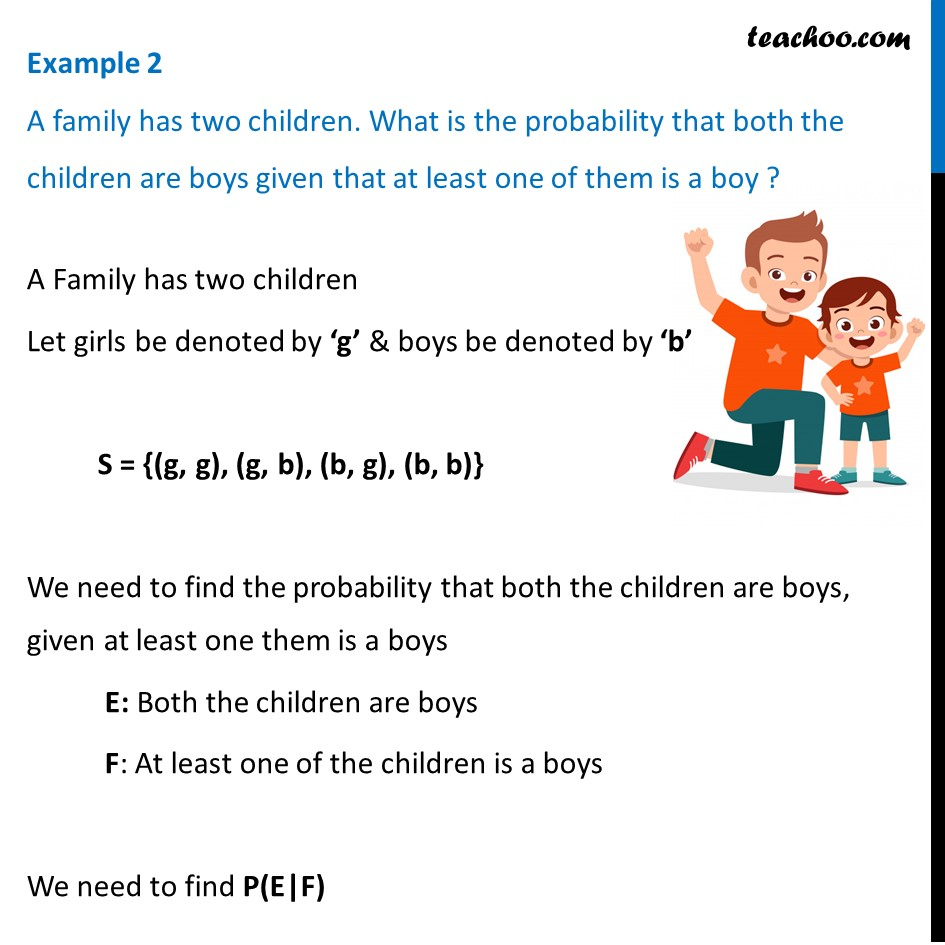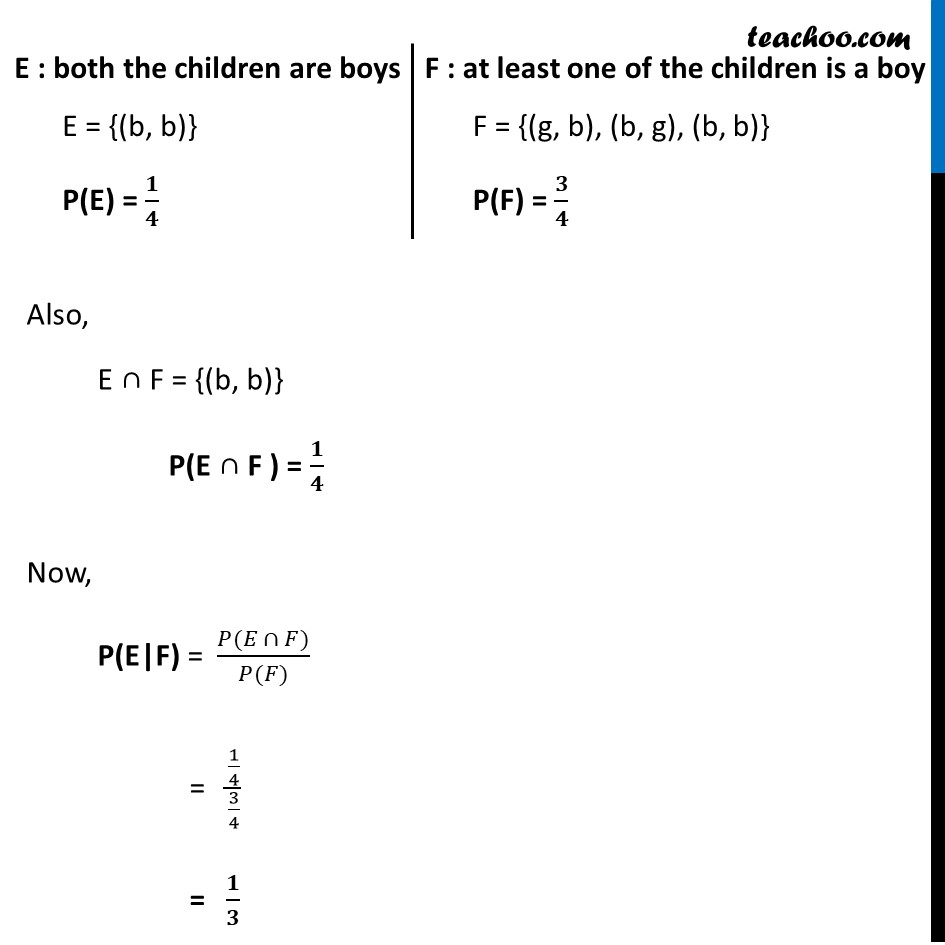Examples

Chapter 13 Class 12 Probability
Serial order wiseLearn in your speed, with individual attention - Teachoo Maths 1-on-1 Class

### Transcript

Example 2 A family has two children. What is the probability that both the children are boys given that at least one of them is a boy ? A Family has two children Let girls be denoted by ‘g’ & boys be denoted by ‘b’ S = {(g, g), (g, b), (b, g), (b, b)} We need to find the probability that both the children are boys, given at least one them is a boys E: Both the children are boys F: At least one of the children is a boys We need to find P(E|F) E : both the children are boys E = {(b, b)} P(E) = 𝟏/𝟒 F : at least one of the children is a boy F = {(g, b), (b, g), (b, b)} P(F) = 𝟑/𝟒 Also, E ∩ F = {(b, b)} P(E ∩ F ) = 𝟏/𝟒 Now, P(E|F) = (𝑃(𝐸 ∩ 𝐹))/(𝑃(𝐹)) = (1/4)/(3/4) = 𝟏/𝟑